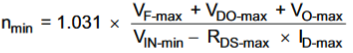• Resolved

# SN6505B: SN6505 Transformer Turns Ratio Formula

Part Number: SN6505B

I'm trying to use the SN6505B to isolate power from USB2.0 (5V). I have a switch and LDO on the secondary side with a maximum input voltage of 5.5V. Given the USB output voltage ranges of 4.4V min and 5.25V max, I want to adjust the turns ratio on the transformer to deliver less than 5.5V to the switch and LDO and at the same time meet the minimum input voltage of the LDO to keep it in regulation.

I've gone through the formula in the datasheet to calculate the minimum turns ratio, but I have a doubt about it. As expected the number of turns on the primary needs to be higher than the secondary to step down the input voltage. It's the 1.031 in the formula that gives me pause since it is not really explained.

nmin = 1.031 * (0.2 + 0.2 + 3.3) / (4.4 - 0.25*1) = 0.919

My questions are: 1. Does my calculation look accurate? 2. What should I make of the 1.031 in the formula?

Gus Martinez

• Hi Gus,

Thank you for posting to E2E! To stay within a maximum 5.5V output, transformers with N = 1:1 turns ratios (like Pulse's PH9085.011NL) are available to use if the maximum input voltage will not exceed 5.25V.

Addressing both of your questions, thank you for including this equation: the calculation looks accurate so long as each value lines up with variables in the datasheet equation as copied below.The 1.031 constant included above is a correction factor (constant number) accounting for a typical 96.9% efficiency of off-the-shelf push-pull isolation transformers. This is not a correction for tolerance in turn ratios, but it helps provide conservative turns ratio requirements.